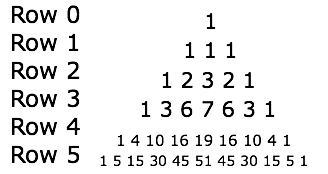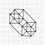# Another Combinations Triangle?

We all know pascal's triangle, right? It's a triangle formed by adding up the two numbers above it. The values it gives form the basis for the famous formula for combinations ->

$$\dbinom{n}{k} = \frac{n!}{k!(n-k)!}$$

So what if we made another type of triangle formed by adding up the three numbers above it?I was contemplating this and have two questions for you:

1. Using the same notation as for Pascal's Triangle , can you make a formula that will take two numbers (r, c), where r is the Row and c is the Column and output the number that appears there in this triangle?

(For example, the 7 in the picture appears at (3,3))

2. Does this triangle represent anything relating to combinations/algebra? Does it have any practical applications?Note by Alex Li
5 years, 1 month ago

This discussion board is a place to discuss our Daily Challenges and the math and science related to those challenges. Explanations are more than just a solution — they should explain the steps and thinking strategies that you used to obtain the solution. Comments should further the discussion of math and science.

When posting on Brilliant:

• Use the emojis to react to an explanation, whether you're congratulating a job well done , or just really confused .
• Ask specific questions about the challenge or the steps in somebody's explanation. Well-posed questions can add a lot to the discussion, but posting "I don't understand!" doesn't help anyone.
• Try to contribute something new to the discussion, whether it is an extension, generalization or other idea related to the challenge.

MarkdownAppears as
*italics* or _italics_ italics
**bold** or __bold__ bold
- bulleted- list
• bulleted
• list
1. numbered2. list
1. numbered
2. list
Note: you must add a full line of space before and after lists for them to show up correctly
paragraph 1paragraph 2

paragraph 1

paragraph 2

[example link](https://brilliant.org)example link
> This is a quote
This is a quote
    # I indented these lines
# 4 spaces, and now they show
# up as a code block.

print "hello world"
# I indented these lines
# 4 spaces, and now they show
# up as a code block.

print "hello world"
MathAppears as
Remember to wrap math in $$ ... $$ or $ ... $ to ensure proper formatting.
2 \times 3 $2 \times 3$
2^{34} $2^{34}$
a_{i-1} $a_{i-1}$
\frac{2}{3} $\frac{2}{3}$
\sqrt{2} $\sqrt{2}$
\sum_{i=1}^3 $\sum_{i=1}^3$
\sin \theta $\sin \theta$
\boxed{123} $\boxed{123}$

Sort by:

One of the reasons Pascal's Triangle relates to combinatorics, is that we add two adjacent numbers to get the next. For example, in the row, "14641", the first 4 represents how many ways to choose one person out of 4, and the 6 represents how many ways to choose 2 people out of 4. The first 10 in the next row represents how many ways to choose 2 people out of 5. This makes sense, because whenever we choose a combination of 5 people, either 1 or 2 of the first 4 must be chosen, and the last one is implied.

I will attempt to apply similar ideas/reasoning for this version of Pascal's Triangle. However, I do not think this relates to combinatorics because every person can be in or out, making just 2 possibilities. However, as we add 3 numbers here, there should be 3 cases. Maybe if we invent a new kind of "choose", where $n\choose{a,b}$ is the number of ways to make a group of size $a$ and size $b$ from a large group of size $n$???

- 5 years, 1 month ago

I do not think you really need 3 different numbers in the new invented "Choose", since you can still base it off of rows and columns. It's not a 3D structure, after all. I considered this idea but it did not seem to work at all. I'm starting to think that it doesn't represent anything at all, and I really don't know how to go about approaching part 1 since any (simple) Pascal's triangle formula proof uses logic that it gets based on the results it produces.

- 5 years ago

2) Yes. Think about why Pascal's triangle represents coefficients of $(x+1)^n$. The triangle that you created are the coefficients of some similar expression.

1) See above.

Staff - 5 years, 1 month ago

@Calvin Lin. I inductively figured it was (x^2+x+1)^n but what are the applications of this?

- 5 years ago

@Alex Li. It appears to be the coefficients of (x^2+x+1)^n.

- 5 years ago

Oh, I figured that out thanks to Calvin, but I'm more curious about if these numbers could represent some sort of scenario that could be written in a sentence, like "number of ways to choose r marbles from a bag when c are identical" (though that obviously doesn't work) The answer I mostly want to know is if there is a formula to produce the numbers.

- 5 years ago

So I reattempted this problem and I now have a slightly satisfying result.

Consider row r and column 0. Clearly, there is only 1 solution.

Now, consider row r and column 1. Again, there are r solutions. But why? If you think about the paths to get to this point, they are all downwards except for one point at which it is to the right. There are exactly r different places that this can happen, so there are $\dbinom{n}{1}$ = n ways.

Next, consider row r and column 2. By the last argument, there are $n \choose 2$ ways. But you could also go left once, so you have to add $n \choose 1$.

Now, for row r, column 3. There are $n \choose 2$ ways for down-down-down, and $n \choose 1$ for down-left. However, you could also go left-down, so we multiply by 2, or $2 \choose 1$

Continuing, we find a pattern.

$\sum_{i=0}^{floor(r/2)+1} \dbinom{n}{r-i} * \dbinom{r-i}{i}$ =

$\sum_{i=0}^{floor(r/2)+1} n!/((r-i)!(n-r+i)! * (r-i)!/((r-2i)!i!)$ =

$\sum_{i=0}^{floor(r/2)+1} n!/((n-r+i)!(r-2i)!i!)$ =

OtherCombinationsTriangle(n,r)

That's the furthest I've got to simplifying it. Can anyone do better?

- 4 years, 6 months ago

Seeing that they are the coefficients of $(x^2 + x + 1)^n$ is interesting in itself. There is a lot of literature on the trinomial coefficients, so that might be a way to learn about them.

Unfortunately, I do not think there is necessarily a "simple" expression for them.

Staff - 4 years, 6 months ago